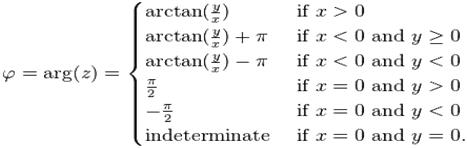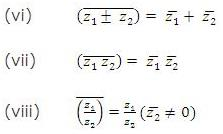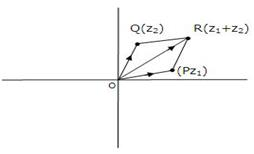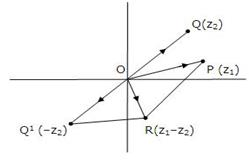Courses

# Notes | EduRev

## JEE : Notes | EduRev

The document Notes | EduRev is a part of the JEE Course Mock Test Series for JEE Main & Advanced 2021.
All you need of JEE at this link: JEE
• z1 = a+ib and z= c+id then z1 = zimplies that a = c and b = d.
• If we have a complex number z where z = a+ib, the conjugate of the complex number is denoted by z* and is equal to a-ib. In fact, for any complex number z, its conjugate is given by z* = Re(z) – Im(z).
• Division of complex numbers: The numerator as well as denominator should first be multiplied by the conjugate of the denominator and then simplified.

Argument of a complex number:
1. Argument of a complex number p(z) is defined by the angle which OP makes with the positive direction of x-axis.
2. Argument of z generally refers to the principal argument of z (i.e. the argument lying in (–π, π) unless the context requires otherwise.
3. Hence, the argument of the complex number z = a + ib = r (cos θ + i sin θ) is the value of θ satisfying r cos θ = a and r sin θ = b.
4. The angle θ is given by θ = tan-1 |b/a|.
5. The value of argument in various quadrants is given below:• If OP = |z| and arg (z) = θ, then obviously z = r (cos θ + i sin θ) and is called the polar form of complex number z.
• |(z-z1)/(z-z2)| =1 the locus of point representing z is the perpendicular bisector of line joining z1 and z2.
• -|z| ≤ Re(z) ≤ |z| and -|z| ≤ Im(z) ≤ |z|
• If a and b are real numbers and zand z2 are complex numbers then
|az1 + bz2|2 + |bz1 - az2|2 = (a2 + b2) (|z1|2 + (|z2|2)
• The distance between the complex numbers z1 and z2 is given by |z- z2|.
• In parametric form, the equation of line joining z1 and z2 is given by z = tz1 + (1-t)z2.
• If A(z1) and B(z2) are two points in the argand plane, then the complex slope μ of the straight line AB is given by μ = (z1 - z2)/ (1 - 2).
• Two lines having complex slopes μ1 and μ2 are:
1. Parallel iff μ= μ2
2. Perpendicular iff μ1 = - μ2 or μ1 + μ= 0
• If A(z1), B(z2), C(z3) and D(z4) are four points in the argand plane, then the angle θ between the lines AB and CD is given by θ = arg{(z1 - z2)/ (z3 – z4)}
• Some basic properties of complex numbers:
I. ||z1| - |z2|| = |z1+z2| and |z1-z2| = |z1| + |z2| iff origin, z1, and z2 are    collinear and origin lies between zand z2.
II. |z1 + z2| = |z1| + |z2| and ||z1| - |z2|| = |z1-z2| iff origin, zand zare collinear and z1 and z2 lie on the same side of origin.
III. The product of nth roots of any complex number z is z(-1)n-1.
IV. amp(zn) = n amp z
V. The least value of |z - a| + |z - b|  is |a - b|.
•Demoivre's Theorem: The theorem can be stated in two forms:
Case I: If n is any integer, then
(i) (cos θ + i sin θ)n = cos nθ + i sin nθ
(ii) (cos θ+ i sin θ1) . (cos θ2 + i sin θ2) ......... (cos θn + i sin θn)
= cos (θ+ θ2 + θ3 .................. + θn) + i sin (θ1 + θ2 + ..............  θn)
Case II: For p and q such that q ≠ 0, we have
(cosθ + isinθ)p/q = cos((2kπ + pq)/q) + isin((2kπ+pq/q)  where k = 0,1,2,3,.....,q-1
• Demoivre’s formula does not hold for non-integer powers.
• Main application of Demoivre’s formula is in finding the nth roots of unity. So, if we write the complex number z in the polar form then,
z = r(cos x + isin x)
Then z1/n = [r (cos x +i sinx )]1/n
= r 1/n [ cos (x+2kπ/n) + i sin (x+2kπ/n)]
Here k is an integer. To get the n different roots of z one only needs to consider values of k from 0 to n –1
• Continued product of the roots of a complex quantity should be determined using theory of equations.
• The modulus of a complex number is given by |z| = √x2+y2.
• The only complex number with modulus zero is the number (0, 0).
• The following figures illustrate geometrically the meaning of addition and subtraction of complex numbers:Offer running on EduRev: Apply code STAYHOME200 to get INR 200 off on our premium plan EduRev Infinity!

## Mock Test Series for JEE Main & Advanced 2021

6 videos|259 docs|164 tests

,

,

,

,

,

,

,

,

,

,

,

,

,

,

,

,

,

,

,

,

,

;